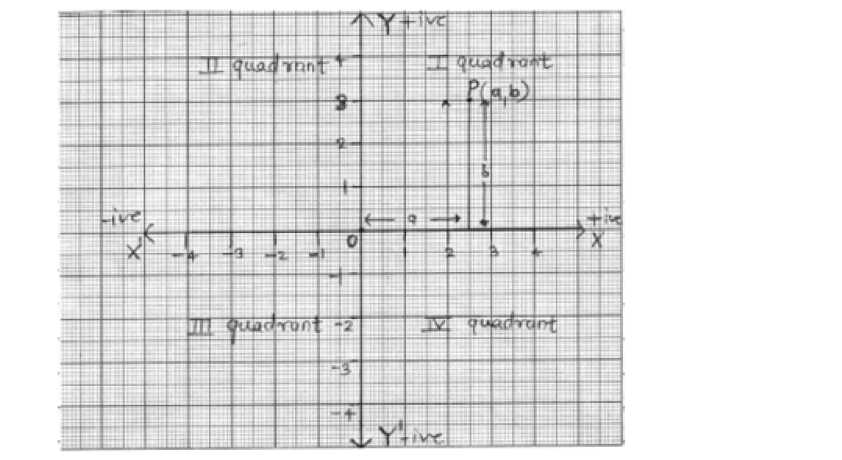# Chapter 3 Coordinate Geometry Notes for Class 9th Maths

0
663

Chapter – 3 Coordinate Geometry

1. Cartesian System
2. Plotting a Point in the Plane with given Coordinates
• Coordinate Geometry: The branch of mathematics in which geometric problems are solved

through algebra by using the coordinate system is known as coordinate geometry.

• Coordinate System: Coordinate axes: The position of a point in a plane is determined with

reference to two fixed mutually perpendicular lines, called the coordinate axes.In this system, position of a point is described by ordered pair of two numbers.

Ordered pair: A pair of numbers a and b listed in a specific order with ‘a’ at the first place and ‘b’ at the second place is called an ordered pair (a,b)

Note that (a,b) ^ (b,a)

Thus (2,3) is one ordered pair and (3,2) is another ordered pair.

In given figure O is called origin.

The horizontal line X1 OX is called the X-axis.

The vertical line YOY’ is called the Y-axis.

P(a,b) be any point in the plane. ‘a’ the first number denotes the distance of point from Y-axis and ‘b’ the second number denotes the distance of point from X-axis. a – X – coordinate | abscissa of P. b – Y – coordinate | ordinate of P.

The coordinates of origin are (0,0)

Every point on the x-axis is at a distance o unit from the X-axis. So its ordinate is 0.

Every point on the y-axis is at a distance of unit from the Y-axis. So, its abscissa is 0.Note: Any point lying on X – axis or Y – axis Y-axis does not lie in any quadrant.